# NEET Previous Year Questions (2014-21): Ray Optics & Optical Instruments Notes | Study Physics Class 12 - NEET

## NEET: NEET Previous Year Questions (2014-21): Ray Optics & Optical Instruments Notes | Study Physics Class 12 - NEET

The document NEET Previous Year Questions (2014-21): Ray Optics & Optical Instruments Notes | Study Physics Class 12 - NEET is a part of the NEET Course Physics Class 12.
All you need of NEET at this link: NEET

Q.1. A convex lens A of focal length 20 cm and a concave lens B of focal length 5 cm are kept along the same axis with the distance d between them. If a parallel beam of light falling on A leaves B as a parallel beam, then distance d in cm will be      (2021)
A: 25
B: 15
C: 30
D: 50

Ans: B
In the absence of concave lens the parallel beam will be focussed at F2 i.e. at a distance 20 cm. from lens A.
The focal length of concave lens is 5 cm. i.e. if this lens is placed at 5 cm from F2 , then beam will become parallel. So d = 15 cm.Q.2. Find the value of the angle of emergence from the prism. The refractive index of the glass is √3.     (2021)A: 45°
B: 90°
C: 60°
D: 30°

Ans: Cr1 + r2 = A = 30°
r2 = 30° (r1 = 0°)
From Snell's law,
√3sinr2 = 1 x sine
√3sin30° = sine
e = 60°

Q.3. A lens of large focal length and large aperture is best suited as an objective of an astronomical telescope since:     (2021)
A: a large aperture contributes to the quality and visibility of the images.
B:
a large area of the objective ensures better light-gathering power.
C: a large aperture provides a better resolution.
D: all of the above.

Ans: D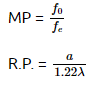Large aperture(a) of the objective lens provides better resolution
∴ Good quality of image is formed and also it gathers more light.

Q.4. A point object is placed at a distance of 60 cm from a convex lens of focal length 30 cm. If a plane mirror were put perpendicular to the principal axis of the lens and at a distance of 40 cm from it, the final image would be formed at a distance of:     (2021)A: 30 cm from the plane mirror, it would be a virtual image.
B: 20 cm from the plane mirror, it would be a virtual image.
C: 20 cm from the lens, it would be a real image.
D: 30 cm from the lens, it would be a real image.

Ans: B
first, for image formation from lens
u = – 60 cm
f = + 30 cm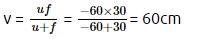this real image formed by lens acts as virtual object for mirrorReal image from plane mirror is formed 20 cm in front of mirror, hence at 20 cm distance from lens.
Now,  for second refraction from lens,
u = – 20 cm
f = +30 cm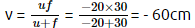So,  Final virtual image is 60 cm from lens, or 20 cm behind mirror.Q.5. A ray is incident at an angle of incidence i on one surface of a small angle prism (with angle of prism A) and emerges normally from the opposite surface. If the refractive index of the material of the prism is m, then the angle of incidence is nearly equal to:     (2020)
A: μA
B: μA/2
C: A/2μ
D: 2A/μ

Ans: A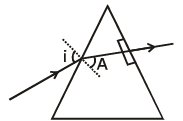1 sin i = μ. sin A
for small angle approximation
sin θ = θ
i = μA

Q.6. Light with an average flux of 20 W/cm2 falls on a non-reflecting surface at normal incidence having surface area 20 cm2. The energy received by the surface during time span of 1 minute is:     (2020)
A: 24 × 103 J
B: 48 × 103 J
C: 10 × 103 J
D: 12 × 103 J

Ans: A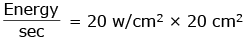= 400 w
∴ in 1 minute = 400 × 60
= 24000

Q.7. Pick the wrong answer in the context with rainbow.    (2019)
A: When the light rays undergo two internal reflections in a water drop, a secondary rainbow is formed
B: The order of colours is reversed in the secondary rainbow
C: An observer can see a rainbow when his front is towards the sun
D: Rainbow is a combined effect of dispersion refraction and  reflection of sunlight
Ans:
C
Solution:

Rainbow can't be observed when observer faces towards sun.

Q.8. Two similar thin equi-convex lenses, of focal length f each, are kept coaxially in contact with each other such that the focal length of the combination is F1. When the space between the two lenses is filled with glycerine (which has the same refractive index (μ = 1.5) as that of glass) then the equivalent focal length is F2. The ratio F1 :  F2 will be :    (2019)
A: 2 : 1
B: 1 : 2
C: 2 : 3
D: 3 : 4
Ans:
B
Solution: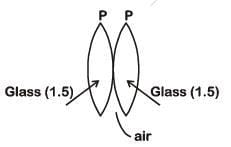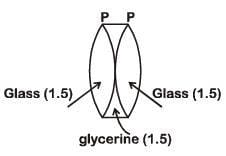Equivalent focal length in air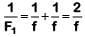When glycerin is filled inside, glycerin lens behaves like a diverging lens of focal length (-f)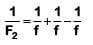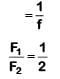Q.9. In total internal reflection when the angle of incidence is equal to the critical angle for the pair of media in contact, what will be angle of refraction?    (2019)
A: 180°
B: 0°
C: Equal to angle of incidence
D: 90°
Ans:
D
Solution:

At i = ic, refracted ray  grazes  with the surface.
So angle of refraction is 90°.

Q.10. The refractive index of the material of a prism is √2 and the angle of the prism is 30°. One of the two refracting surfaces of the prism is made a mirror inwards, by silver coating. A beam of monochromatic light entering the prism from the other face will retrace its path (after reflection from the silvered surface) if its angle of incidence on the prism is :-    (2018)
A: 60°
B: 45°
C: 30°
D: zero
Ans:
B
Solution: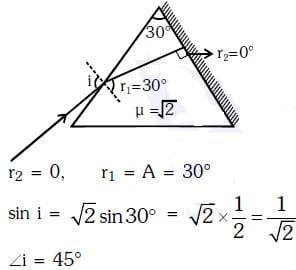Q.11. An object is placed at a distance of 40 cm from a concave mirror of focal length 15 cm. If the object is displaced through a distance of 20 cm to wards the mirror, the displacement of the image will be:-    (2018)
A: 30 cm away from the mirror
B: 36 cm away from the mirror
C: 30 cm towards the mirror
D: 36 cm towards the mirror
Ans:
B
Solution: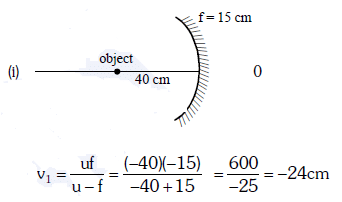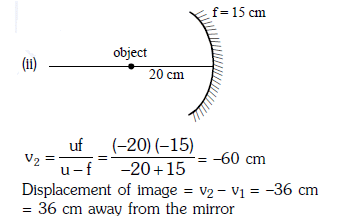Q.12. An astronomical refracting telescope will have large angular magnification and high angular resolution, when it has an objective lens of :-    (2018)
A: small focal length and large diameter
B: large focal length and small diameter
C: large focal length and large diameter
D: small focal length and small diameter
Ans: C
Solution:

For astronomical refracting telescope
Angular magnification is for large focal length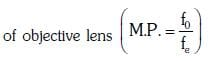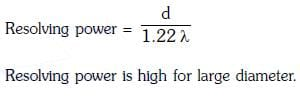Q.13. A beam of light from a source L is incident normally on a plane mirror fixed at a certain distance x from the source. The beam is reflected back as a spot on a scale placed just above the source I. When the mirror is rotated through a small angle q, the spot of the light is found to move through a distance yon the scale. The angle q is given by :-   (2017)
A: y/x
B: x/2y
C: x/y
D: y/2x

Ans: D
Solution: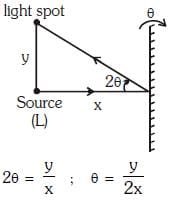Q.14. The ratio of resolving powers of an optical microscope for two wave lengths λ1 = 4000 Å and λ2 = 6000 Å is :-    (2017)
A: 9 : 4
B: 3 : 2
C: 16 : 81
D: 8 : 27
Ans:
B
Solution: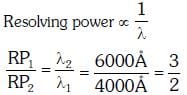Q.15. A thin prism having refracting angle 10° is made of glass of refractive index 1.42. This prism is combined with another thin prism of glass of refractive index 1.7. This combination produces dispersion without deviation. The refracting angle of second prism should be :-    (2017)
A: 6°
B: 8°
C: 10°
D: 4°
Ans:
A
Solution:

(μ -1)A + (μ'-1)A = 0
(μ-1)A |(μ'-1)A'|
(1.42 -1)x10° = (1.7 -1)A'
4.2 = 0.7A'
A' = 6°

Q.16. A astronomical telescope has objective and eyepiece of focal lengths 40 cm and 4 cm respectively. To view an object 200 cm away from the objective, the lenses must be separated by a distance :    (2016)
A: 54 cm
B: 37.3 cm
C: 46 cm
D: 50 cm
Ans: A
Solution:

According to a question,
Focal length of the objective lens, Fo = +40 cm
Focal length of eyepiece, Fe = 4 cm
Object distance for objective lens (uo) = -200 cm
Applying lens formula for objective lens,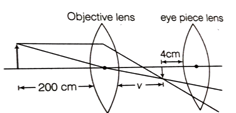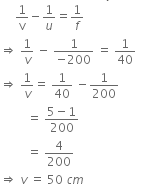Image will be formed at the focus of a eyepiece lens.
So, for normal adjustment distance between objectives and eyepiece (length of tube) will be, v + Fe = 50 + 4 = 54 cm

Q.17. Match the corresponding entries of column-1 with column-2. [Where m is the magnification produced by the mirror]     (2016)
Column - 1                   Column - 2

(A) m = -2              (a) Convex mirror
(B) m = -1/2          (b) Concave mirror
(C) m = +2             (c) Real image
(D) m = =1/2         (d) Virtual image
A: A → c and d; B → b and d; C → b and c; D → a and d
B: A → b and c; B → b and c; C → b and d; D → a and d
C: A → a and c; B → a and d; C → a and b; D → c and d
D: A → a and d; B → b and c; C → b and d; D → b and c
Ans:
B
Solution:

(A) m = -2, so image is magnified and inverted. Which is possible only for concave mirror. since image is i inverted so it will be real.
(B) M = -1/2 , so image is inverted and diminished. since image is inverted, so it will be real, and the mirror will be concave.
(C) M = +2, image is magnified so the mirror will be concave. Image is erect so it will be virtual.
(D) m = +1/2 , image is erect so image will be virtual. Image is virtual and diminished, so the mirror should be convex.

Q.18. The angle incidence for a ray of light at a refracting surface of a prism is 45 degree.The angle of prism is 60 degree If the ray suffers minimum deviation through the prism, the angle of minimum deviation and refractive index of the material of the prism respectively, are     (2016)
A: 30 deg; 1/√2
B: 45 deg; 1/√2
C: 30 deg; √2
D: 45 deg; √2
Ans:
C
Solution: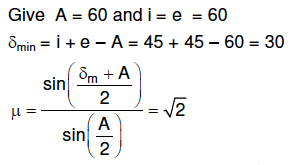Q.19.  The refracting angle of a prism is A, and refractive index of the material of the prism is cot (A/2). The angle of minimum deviation is :     (2015)
A: 180º + 2A
B: 180º - 3A
C: 180º - 2A
D: 90º - A
Ans:
C
Solution: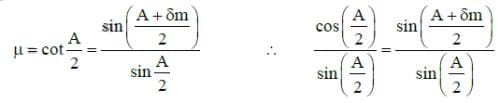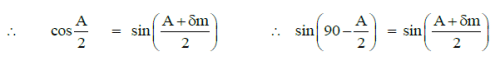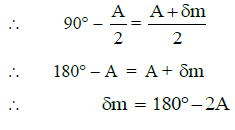Q.20. Two identical thin plano-convex glass lenses (refractive index 1.5) each having radius of curvature of 20 cm are placed with their convex surfaces in contact at the centre. The intervening space is filled with oil of refractive index 1.7. The focal length of the combination is :     (2015)
A: 50 cm
B: -20 cm
C: -25 cm
D: -50 cm
Ans:
D
Solution: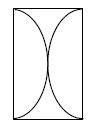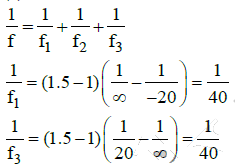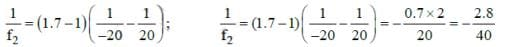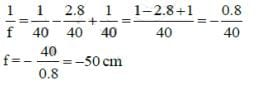Q.21. The angle of a prism is ‘A’. One of its refracting surfaces is silvered. Light rays falling at an angle of incidence 2A on the first surface returns back through the same path after suffering reflection at the silvered surface. The refractive index μ, of the prism is :     (2014)
A: 1/2 cos A
B: tan A
C: 2 sin A

D: 2 cos A
Ans:
D
Solution: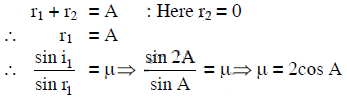Q.22. If the focal length of objective lens is increased then magnifying power of :      (2014)
A: microscope and telescope both will decrease
B: microscope will decrease but that of telescope will increase
C: microscope will increase but that of telescope will decrease
D: microscope and telescope both will increase
Ans:
B
Solution: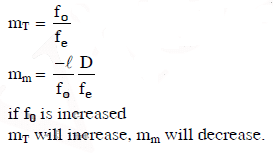The document NEET Previous Year Questions (2014-21): Ray Optics & Optical Instruments Notes | Study Physics Class 12 - NEET is a part of the NEET Course Physics Class 12.
All you need of NEET at this link: NEETUse Code STAYHOME200 and get INR 200 additional OFF

## Physics Class 12

134 videos|388 docs|213 tests

### How to Prepare for NEET

Read our guide to prepare for NEET which is created by Toppers & the best Teachers

Track your progress, build streaks, highlight & save important lessons and more!

,

,

,

,

,

,

,

,

,

,

,

,

,

,

,

,

,

,

,

,

,

;